CAT  >  Practice Questions Level 1: Geometry - 2

# Practice Questions Level 1: Geometry - 2 - Notes | Study Level-wise Practice Questions for CAT Preparation - CAT

 1 Crore+ students have signed up on EduRev. Have you?

This EduRev document offers 20 Multiple Choice Questions (MCQs) from the topic Geometry (Level - 1). These questions are of Level - 1 difficulty and will assist you in the preparation of CAT & other MBA exams. You can practice/attempt these CAT Multiple Choice Questions (MCQs) and check the explanations for a better understanding of the topic.

Question for Practice Questions Level 1: Geometry - 2
Try yourself:A vertical stick 20 m long casts a shadow 10 m long on the ground. At the same time, a tower casts the shadow 50 m long on the ground. Find the height of the tower.

Question for Practice Questions Level 1: Geometry - 2
Try yourself:The area of similar triangles, ABC and DEF are 144 cm2 and 81 cm2 respectively. If the longest side of larger ΔABC be 36 cm, then the longest side of smaller ΔDEF is

Question for Practice Questions Level 1: Geometry - 2
Try yourself:The areas of two similar Ds are respectively 9 cm2 and 16 cm2. Find the ratio of their corresponding sides.

Question for Practice Questions Level 1: Geometry - 2
Try yourself:If O is the centre of circle, find ∠x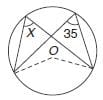Question for Practice Questions Level 1: Geometry - 2
Try yourself:Find the value of x in the given figure.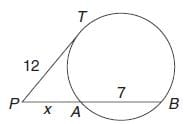Question for Practice Questions Level 1: Geometry - 2
Try yourself:In the given figure, find ∠ADB.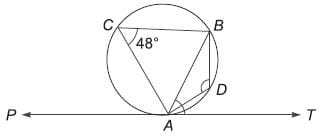Question for Practice Questions Level 1: Geometry - 2
Try yourself:In the following figure, O is the centre of the circle and ∠ABO = 30°, find ∠ACB.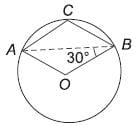Question for Practice Questions Level 1: Geometry - 2
Try yourself:In the figure, AB is parallel to CD and RD | | SL || TM || AN, and BR : RS : ST : TA = 3 : 5 : 2 : 7. If it is known that CN = 1.333 BR. Find the ratio of BF : FG : GH : HI : IC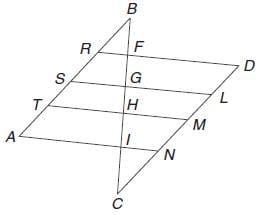Question for Practice Questions Level 1: Geometry - 2
Try yourself:In the figure, DABC is similar to DEDC.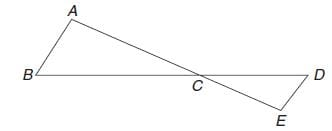If we have AB = 4 cm,
ED = 3 cm, CE = 4.2 and
CD = 4.8 cm, find the value of CA and CB

Question for Practice Questions Level 1: Geometry - 2
Try yourself:Two isosceles Ds have equal angles and their areas are in the ratio 16 : 25. Find the ratio of their corresponding heights.

Question for Practice Questions Level 1: Geometry - 2
Try yourself:Two poles of height 6 m and 11 m stand vertically upright on a plane ground. If the distance between their foot is 12 m, find the distance between their tops.

Question for Practice Questions Level 1: Geometry - 2
Try yourself:Find the length of a chord that is at a distance of 12 cm from the centre of a circle of radius 13 cm.

Question for Practice Questions Level 1: Geometry - 2
Try yourself:Find the value of ∠x in the given figure.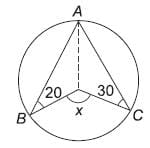Question for Practice Questions Level 1: Geometry - 2
Try yourself:Find the value of x in the given figure.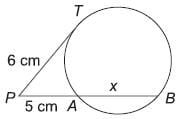Question for Practice Questions Level 1: Geometry - 2
Try yourself:Find the value of x in the given figure.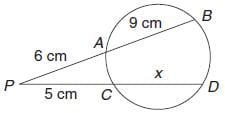Question for Practice Questions Level 1: Geometry - 2
Try yourself:In the given figure AB is the diameter of the circle and ∠PAB = 25°. Find ∠TPA.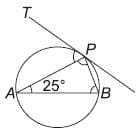Question for Practice Questions Level 1: Geometry - 2
Try yourself:In the given figure, two straight lines PQ and RS intersect each other at O. If ∠SOT = 75°, find the value of a, b and c.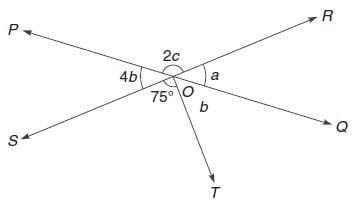Question for Practice Questions Level 1: Geometry - 2
Try yourself:In the following figure, it is given that o is the centre of the circle and ∠AOC = 140°. Find ∠ABC.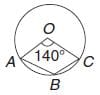Question for Practice Questions Level 1: Geometry - 2
Try yourself:In the following figure, find the value of x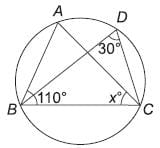Question for Practice Questions Level 1: Geometry - 2
Try yourself:Find the perimeter of the given figure.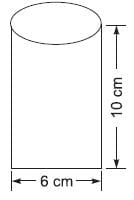The document Practice Questions Level 1: Geometry - 2 - Notes | Study Level-wise Practice Questions for CAT Preparation - CAT is a part of the CAT Course Level-wise Practice Questions for CAT Preparation.
All you need of CAT at this link: CAT

## Level-wise Practice Questions for CAT Preparation

277 docs
 Use Code STAYHOME200 and get INR 200 additional OFF

## Level-wise Practice Questions for CAT Preparation

277 docs

### How to Prepare for CAT

Read our guide to prepare for CAT which is created by Toppers & the best Teachers

Track your progress, build streaks, highlight & save important lessons and more!

,

,

,

,

,

,

,

,

,

,

,

,

,

,

,

,

,

,

,

,

,

;